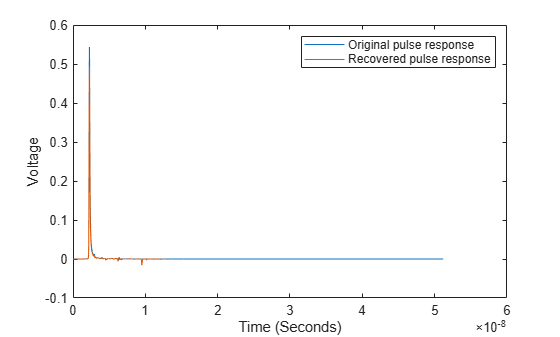wave2pulse

Pulse response from data pattern waveform

Description

example

P = wave2pulse(W,D,N) converts a data pattern waveform W to a pulse response P, given the symbol pattern D and the number of samples per symbol N.

P = wave2pulse(___,K) converts a data pattern waveform to a truncated pulse response so that the first dimension of P is of size (N·K), where K is the desired length of the pulse response.

Examples

collapse all

Load pulse response column matrix from a file.

Select the primary pulse response and remove any DC components.

P1 = pulse(:,1) - pulse(1,1);

Create a symbol pattern of PRBS order 7.

order = 7;
data = prbs(order,2^order-1)-0.5;

Create a data pattern waveform from the pulse response.

W1 = pulse2wave(P1,data,SamplesPerSymbol);

Apply memoryless nonlinearity to the waveform using a serdes.SaturatingAmplifier object. The saturating amplifier clips any voltage over 0.4 V.

SatAmp = serdes.SaturatingAmplifier('Limit',0.4);
W2 = SatAmp(W1);

Recover the pulse response from the waveform W2.

P2 = wave2pulse(W2,data,SamplesPerSymbol);

Plot the original and recovered pulse responses.

t2 = dt*(0:length(P2)-1);
figure
plot(t,P1,t2,P2)
xlabel('Time (Seconds)')
ylabel('Voltage')
legend('Original pulse response','Recovered pulse response')Input Arguments

collapse all

Data pattern waveform, specified as a column vector.

Data Types: double

Symbol pattern contained within the data pattern waveform W, specified as a vector.

Data Types: double

Number of samples per symbol, specified as a positive integer scalar.

Data Types: double

Desired length of the pulse response, specified as a positive integer scalar in symbols.

Data Types: double

Output Arguments

collapse all

Converted pulse response, returned as a column matrix. The first column contains the primary pulse response and the subsequent columns (if any) contain the crosstalk pulse responses.

Data Types: double

Extended Capabilities

C/C++ Code GenerationGenerate C and C++ code using MATLAB® Coder™.